Clutch Prep is now a part of Pearson
Ch.3 - Chemical ReactionsWorksheetSee all chapters

# Balancing Chemical Equations Worksheet (IGNORE)

See all sections
Sections
Empirical Formula
Molecular Formula
Combustion Analysis
Combustion Apparatus
Polyatomic Ions
Naming Ionic Compounds
Writing Ionic Compounds
Naming Ionic Hydrates
Naming Acids
Naming Molecular Compounds
Balancing Chemical Equations
Stoichiometry
Limiting Reagent
Percent Yield
Mass Percent
Functional Groups in ChemistryJules Bruno

Under the Law of Conservation of Mass, matter cannot be created nor destroyed and only change form. Balancing a chemical equation ensures this law is followed by having the same number of reactant atoms and product atoms.

Examining a Balanced Equation

In a typical balanced equation the numbers in blue are called your coefficients and represent the number of moles for each compound shown.Coefficients and Balanced Equation

This written chemical reaction tells us that “2 moles of hydrogen, H­, react with 1 mole of oxygen , O­2 , to produce 2 moles of water, H2O. ”

The numbers in red are the subscripts and combined with the coefficients give us the number of each particular element.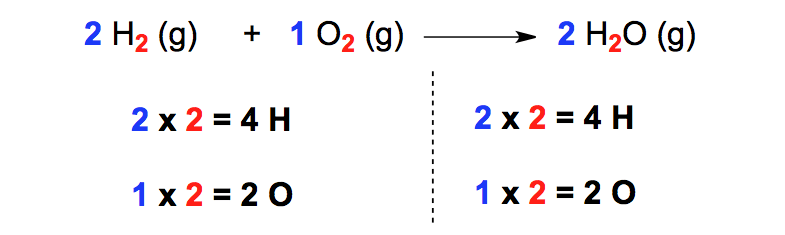Balanced Chemical Reaction

Balancing a Chemical Equation

Just follow our methods for balancing each of the following chemical equations and you’ll be an expert in no time.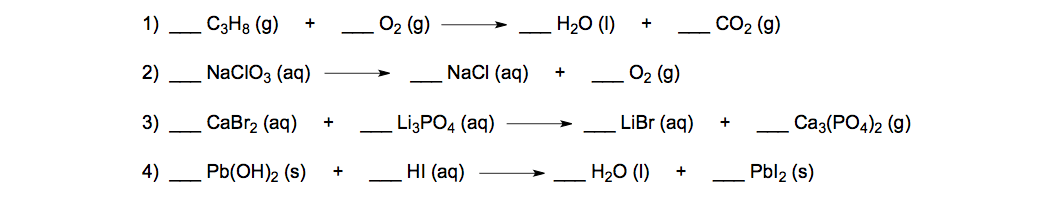Unbalanced Chemical Equations

For all the equations you will need to set up two lists of elements: one for the reactants and another for the products. If your numerical values in both lists don’t match then you’ll have to balance the chemical equation.

Chemical Equation #1

STEP 1: Set up a list for the elements that are reactants and another list for the elements that are products. The two lists don’t match so we will need to balance the chemical equation.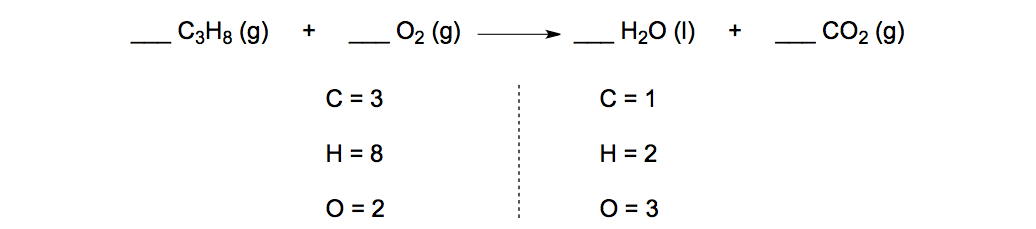Traditional Balance

STEP 2: Start at the top and going down both lists make sure to balance each element one by one.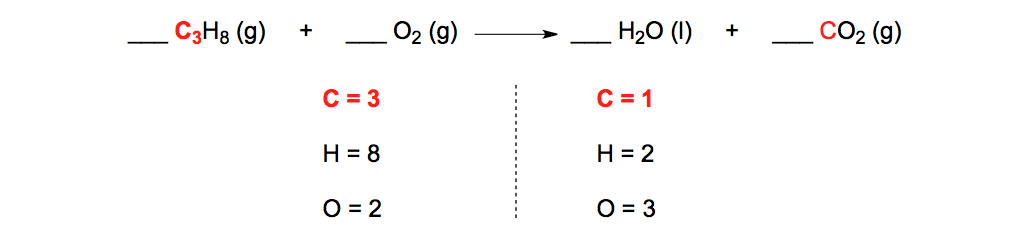Balancing carbon atoms

STEP 3: Balance the number of carbon atoms by placing a “3” in front of CO­2. The “3” is distributed so the number of carbon and oxygen atoms are both affected.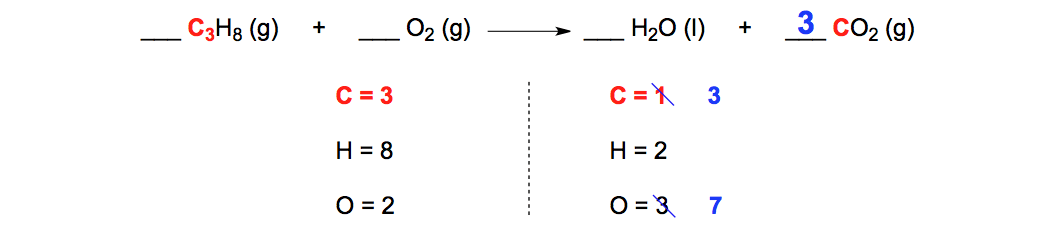Algebraic Balance (Carbon Atoms)

STEP 4: Continuing down the lists we next balance the number of hydrogen atoms. We do this by placing a “4” in front of H­2O that it distributed to hydrogen and oxygen atom.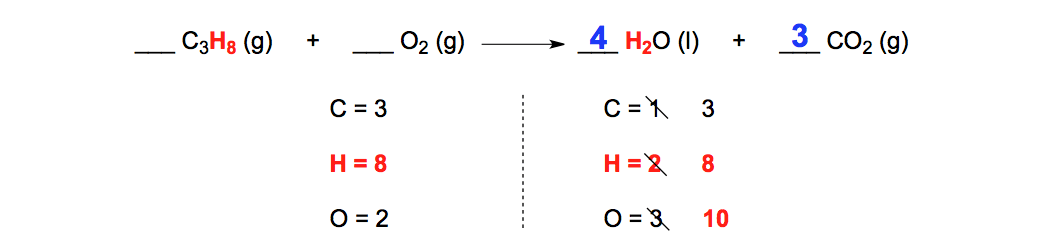Algebraic Balance (Hydrogen Atoms)

STEP 5: Finally balance balance the oxygen atoms by placing a “5” in front of O2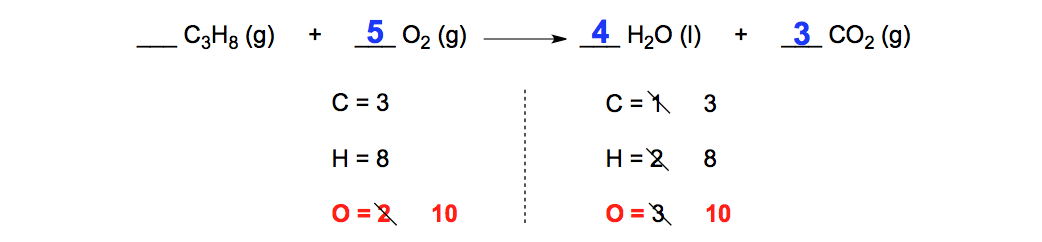Algebraic Balance (Oxygen Atoms)

Chemical Equation #2

STEP 1: Set up your two lists of the elements. Start at the top of the list and work your way down both lists to balance the chemical reaction.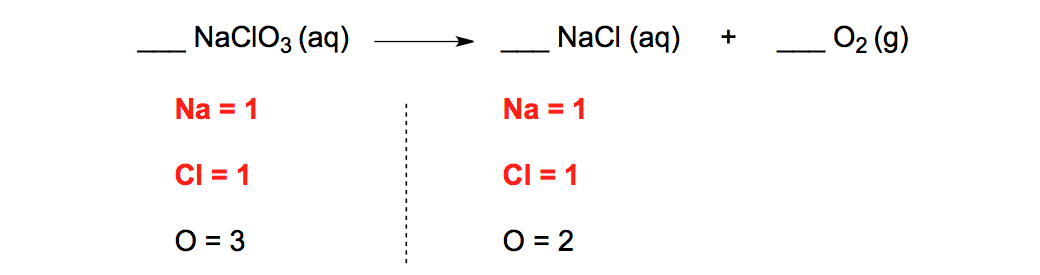Unbalanced Chemical Equation

Since both the sodium and chlorine atoms match we continue to the oxygen atoms.

STEP 2: To balance the oxygen atoms we place a “2” in front of NaClO3 and a “3” in front of O2. This gives “6” oxygen atoms on both sides of the chemical equation.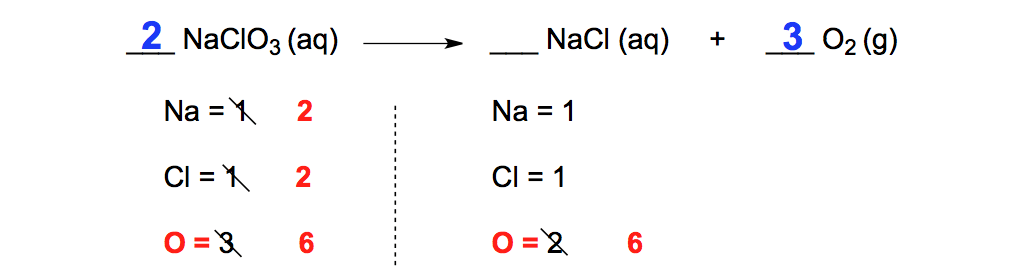Algebraic Balance (Oxygen Atoms)

The “2” in front of NaClO3 is also distributed to the sodium (Na) and chlorine (Cl) atoms. This means they will have to be balanced as well.

STEP 3: To balance the sodium (Na) and chlorine (Cl) atoms we place a “2” in front of NaCl.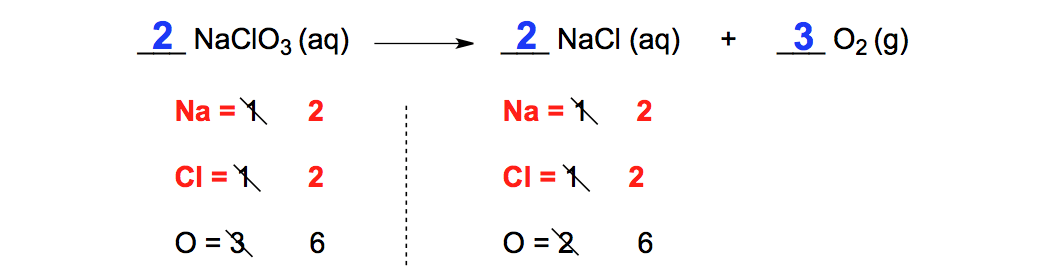Balanced Decomposition Equation

Chemical Equation #3

At times you will have polyatomic ions (SO42-, NH4+, PO43-) that look the same on both sides of the chemical equation. From the chemical equation we have PO4 on both sides and to make balancing easier just place PO4 in your list of atoms.

STEP 1: We need to balance the calcium atoms by placing a “3” in front of CaBr2. The “3” will be distributed to both the calcium and bromine atoms in CaBr2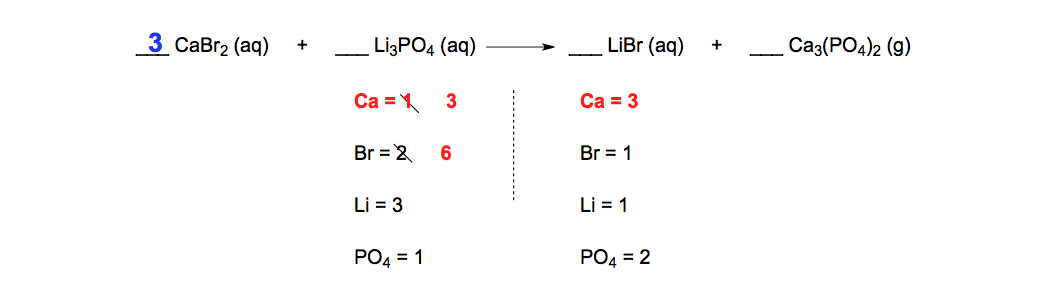Algebraic Balance (Calcium Atoms)

STEP 2: Balance the bromine atoms by placing a “6” in front of LiBr. The “6” will be distributed to both the lithium and bromine atoms.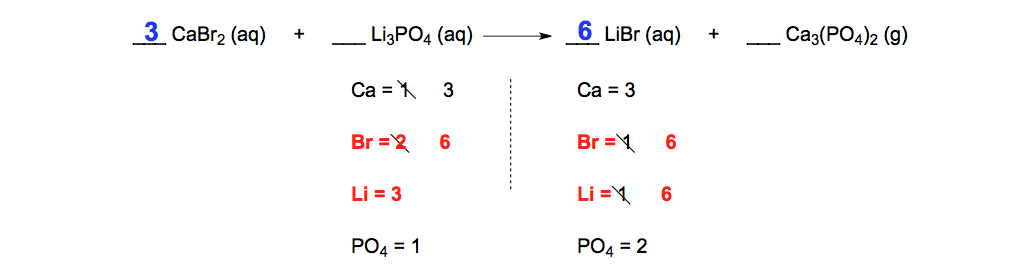Algebraic Balance (Bromine Atoms)

STEP 3: Balance the lithium and PO4 atoms by placing a “2” in front of Li3PO4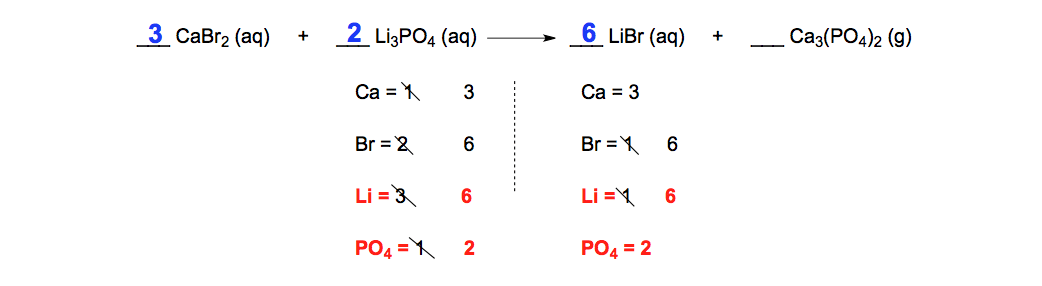Algebraic Balance (Lithium Atoms)

Chemical Equation #4

STEP 1: The lead (Pb) atoms match so we continue to the oxygen atoms.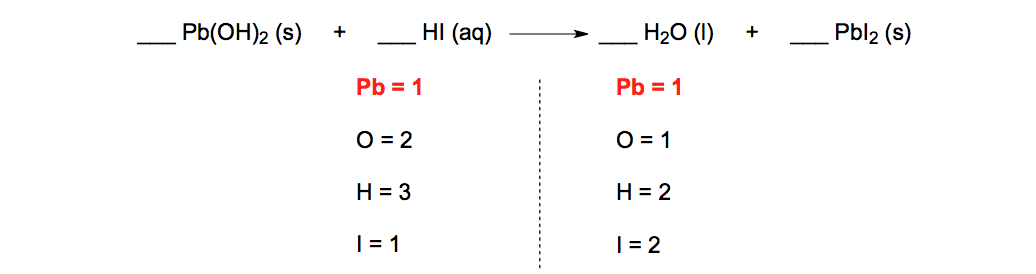Algebraic Balance (Lead Atoms)

The lead (Pb) atoms match so we continue to the oxygen atoms.

STEP 2: Balance the oxygen atoms by placing a “2” in front of H2O. The “2” will be distributed to both the hydrogen and oxygen atoms.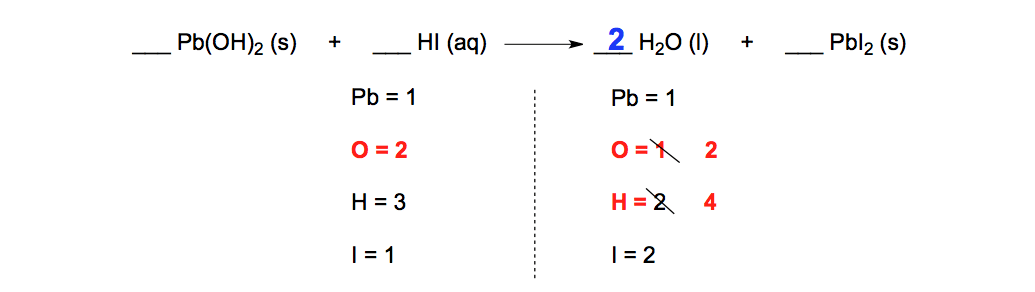Algebraic Balance (Oxygen Atoms)

STEP 3: Balance the hydrogen and iodine atoms by placing a “2” in front of HI.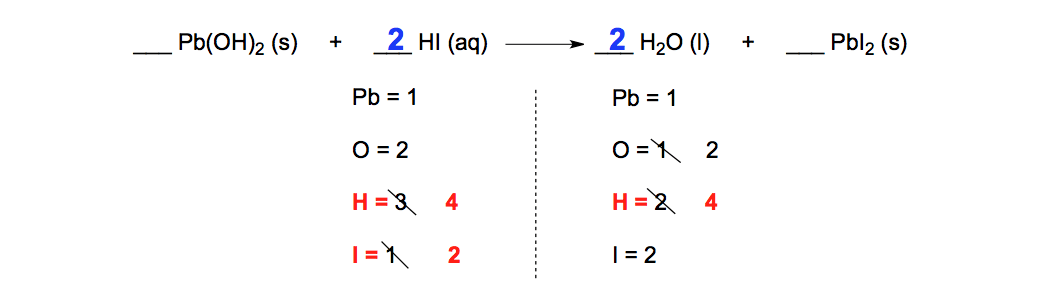Algebraic Balance (Hydrogen and Iodine Atoms)Jules Bruno

Jules felt a void in his life after his English degree from Duke, so he started tutoring in 2007 and got a B.S. in Chemistry from FIU. He’s exceptionally skilled at making concepts dead simple and helping students in covalent bonds of knowledge.